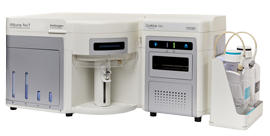### "How many events do you need to acquire?"

Assay variation can be a major source of error, therefore it is critical to understand the statistics behind rare event analysis. The cytometry friendly Gaussian distribution cannot be applied to the analysis of such a low number of events (the term "rare" is given to events with a frequency of 0.01% or less) and the best approach is to use Poisson statistics (Fig.1). Good experimental practice suggests to keep Coefficient of Variation (CV) below 5%, and thus the number of events to acquire should be defined in order to maintain the lowest CV possible and in any case below 5%.

The number of events that satisfy a given criterion (e.g they are positive for a marker, P) is defined as
\$P=R/N\$
where
\$N\$=total events
\$R\$=events that meet a given criterion
and
\$0<=P<=1\$
As with all statistical distribution,
the variance (V) is defined as
\$V(R) = NP(1-P)\$
The standard deviation (SD) is
\$SD=√V=√(NP(1-P))\$
and the coefficient of variation is
\$CV=1/√V\$
Figure 1. Poisson statistics defines the probability that a number of events will occur in a fixed interval of time/space/volume.
Use the tool below to help define the ideal number of events to acquire for the expected frequency of rare population.

Step 1: Input the expected frequency in the cell below; i.e. to identify a cell population that represents 0.01% of the total, input frequency is 0.001 (one positive event every ten thousands)
The number of events that satisfy a given criterion (e.g they are positive for a marker, P) is defined as
\$P=R/N\$
where
\$N\$=total events
\$R\$=events that meet a given criterion
and
\$0<=P<=1\$
As with all statistical distribution,
the variance (V) is defined as
\$V(R) = NP(1-P)\$
The standard deviation (SD) is
\$SD=√V=√(NP(1-P))\$
and the coefficient of variation is
\$CV=1/√V\$
Figure 1. Poisson statistics defines the probability that a number of events will occur in a fixed interval of time/space/volume.
Step 2: Use the Events (N) cells below to input the total number of events, CV% cells will automatically refresh allowing the identification of the ideal total events number or the interval required to achieve a CV below 5%; i.e. to identify a cell population that represents 0.01%, five million events should be acquired.
 Events (N) Positive (R) 0 0 0 0 0 0 Proportion (P) 0 0 0 0 0 0 Variance (V) 0 0 0 0 0 0 SD 0 0 0 0 0 0 CV% 0 0 0 0 0 0
Where:
 Number of events \$\$N\$\$ Positive events \$\$R\$\$
 Proportion (frequency) \$\$P=R/N\$\$ Variance \$\$V=NP(1-P)\$\$
 Standard Deviation \$\$SD=√V\$\$ Coefficient of Variation % \$\$CV=(SDx100)/R\$\$

### Learn how Attune NxT Flow Cytometer can help you count cells and identify populations.